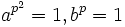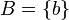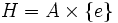# Permutability is not finite-intersection-closed

This article gives the statement, and possibly proof, of a subgroup property (i.e., permutable subgroup) not satisfying a subgroup metaproperty (i.e., finite-intersection-closed subgroup property).
View all subgroup metaproperty dissatisfactions | View all subgroup metaproperty satisfactions|Get help on looking up metaproperty (dis)satisfactions for subgroup properties
Get more facts about permutable subgroup|Get more facts about finite-intersection-closed subgroup property|

## Statement

### Verbal statement

The intersection of two permutable subgroups of a group need not be permutable.

### Symbolic statement

It is possible to find a group$G$ and subgroups$H$ and$K$ of$G$ such that$H$ and$K$ are both permutable subgroups (viz quasinormal subgroups) but$H \cap K$ is not.

## Proof

### Construction of the counterexample

Setup: Let$p$ be an odd prime.

•$A$ is a semidirect product of cyclic group of prime-square order and cyclic group of prime order. More specifically it is a group generated by two elements$a,b$ subject to the relations$a^{p^2} = 1, b^p = 1$ and$ab = ba^{p+1}$. Alternatively$A$ is the semidirect product of the additive group modulo$p^2$ by the multiplicative group of order$p$ in the multiplicative group of automorphisms. Note that$A$ is a non-abelian group of order$p^3$.
•$C$ is a cyclic group of prime-square order: It is a cyclic group of order$p^2$, generated by an element$c$.
•$G = A \times C$.
•$\! B = \{ b \}$.
•$H = A \times \{ e \}$, and$K = B \times C = \{b , c\}$.
•$B_0 = H \cap K = B \times \{ e \}$.

We claim that$H$ and$K$ are both permutable in$G$, but their intersection$B_0 = H \cap K$ is not permutable.

•$H = A \times \{ e \}$ is permutable:$H$ is a direct factor of$G$ so it is clearly a normal subgroup and hence a permutable subgroup.
•$K = B \times C = \{ b,c \}$ is permutable: Since permutability satisfies the inverse image condition, we see that if$B$ is permutable in$A$, then$B \times C = \{ b, c\}$ is permutable in$G$. Thus, it suffices to show that$B$ is permutable as a subgroup of$A$. This can easily be checked by verifying that$B$ commutes with all the cyclic subgroups of$A$. (a proof of this is provided in an example for permutable not implies normal).
•$B_0 = H \cap K = B \times \{ e \}$ is not permutable in$G$: Consider the cyclic subgroup$D$ generated by$(a,c)$. The claim is that$B_0D \ne DB_0$. To prove this notice that$DB_0 \ni (a,c)(b,e) = (ab,c) = (ba^{p+1},c)$. This is clearly not in$B_0D$.

## Further fact shown by the example

This example shows some further facts:

• The intersection of a permutable subgroup with a direct factor need not be a permutable subgroup. In this example, for instance,$A$ is a direct factor, but its intersection with$C$ is still not a permutable subgroup.
• A permutable subgroup of a direct factor need not be a permutable subgroup. In this case$B = A \cap C$ is a permutable subgroup inside$A$, which itself is a direct factor.
• Permutability is not a direct product-closed subgroup property